Trusted answers to developer questions
Trusted Answers to Developer Questions

Related Tags

julia

# What is simple logistic regression in Julia?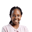Joy Kareko

Grokking Modern System Design Interview for Engineers & Managers

Ace your System Design Interview and take your career to the next level. Learn to handle the design of applications like Netflix, Quora, Facebook, Uber, and many more in a 45-min interview. Learn the RESHADED framework for architecting web-scale applications by determining requirements, constraints, and assumptions before diving into a step-by-step design process.

Logistic Regression is a supervised machine learning algorithm to predict a binary or a multi-class outcome. Read and learn about logistic regression in this Answer here.

We’ll use logistic regression to predict the survival class of people in the titanic dataset and showcase its implementation in Julia.

### Import the necessary libraries

import Pkg
println("Packages successfully imported")


The DataFrames library will be used to read data into a DataFrame, while RDatasets contains the titanic dataset we will use.

The Lathe library will be used to split our dataset into training and testing sets. We’ll use the GLM library to create and train our model and obtain predictions on the test set for evaluation.

Lastly, the StatsBase and MLBase libraries are used to obtain the model’s accuracy and the confusion matrix on our prediction classes.

### Load the dataset and print the first five rows of the data

This dataset is from the COUNT category in RDatasets.

using RDatasets
data = dataset("COUNT", "titanic")
first(data,5)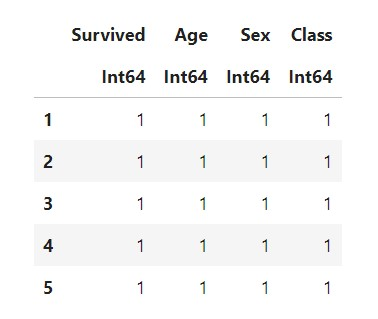### Use describe to check the summary of the dataset.

describe(data)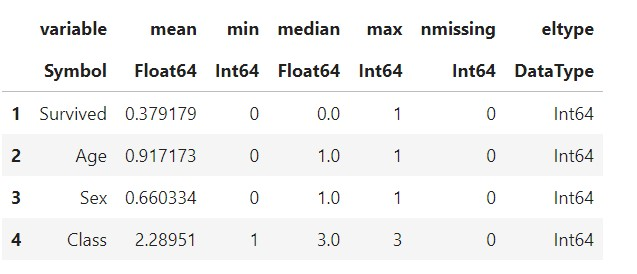There are no missing values, and all our data is of integer type.

### Check the target column, Survived, for imbalance

using StatsBase
countmap(data.Survived)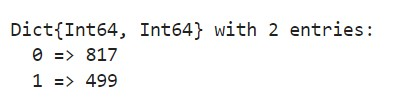Our dataset has a class imbalance, with more data in the Survived Class 0 for non-survivors.

### Split data into test and train tests

using Lathe.preprocess:TrainTestSplit
train, test = TrainTestSplit(data, .80);


The ; at the end is used to suppress the output.

## Train the Logistic Regression Model

using GLM
fm = @formula(Survived ~ Age + Sex + Class)
logit = glm(fm, train, Binomial(), ProbitLink())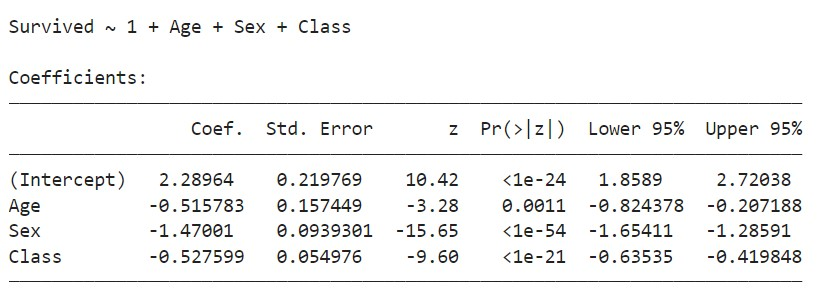This shows the coefficients to the independent variables as well as the intercept. We now have our trained model and our equation.

### Evaluate the model

Predict the target variable on test data

prediction = predict(logit, test);


Convert the probability score to classes.

prediction_class = [if x < 0.5 0 else 1 end for x in prediction];


Have everything in a data frame for ease of comparison.

prediction_df = DataFrame(y_actual = test.Survived,y_predicted = prediction_class,prob_predicted = prediction);


Check correct predictions by comparing them to actual values.

prediction_df.correctly_classified = prediction_df.y_actual .== prediction_df.y_predicted



### Obtaining the accuracy score and confusion matrix

using StatsBase
accuracy = mean(prediction_df.correctly_classified)*100
println("Accuracy is:",accuracy)


We obtain an accuracy score of 75.36%.

Obtaining the confusion matrix:

using MLBase
confusion_matrix = MLBase.roc(prediction_df.y_actual,prediction_df.y_predicted)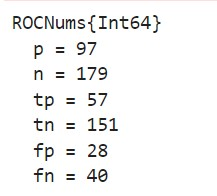Due to class imbalance, our model predicts the majority class better.

Use the terminal below to practice. Type Julia to start.

Terminal 1
Terminal

RELATED TAGS

julia

CONTRIBUTOR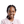Joy Kareko